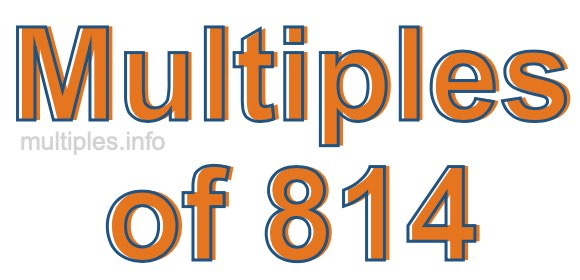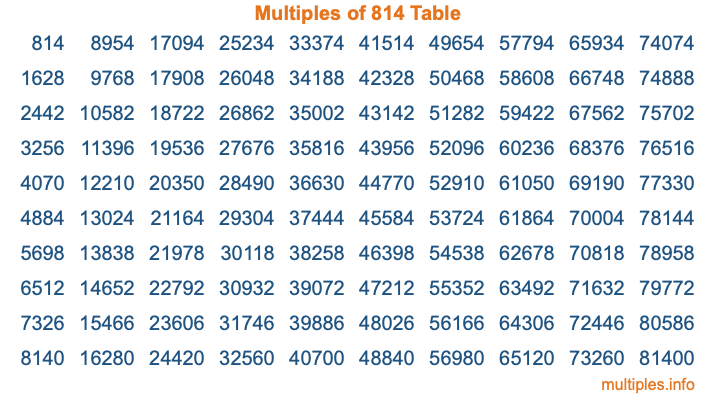Multiples of 814Welcome to the Multiples of 814 page. Here we will first teach you everything you will ever need to know about the multiples of 814, and then give you a study guide summary of everything we taught you to make sure you remember it all. Use this page to look up facts and learn information about the multiples of 814. This page will make you a multiples of eight hundred fourteen expert!

Definition of Multiples of 814
Multiples of 814 are all the numbers that when divided by 814 equal an integer. Each of the multiples of 814 are called a multiple. A multiple of 814 is created by multiplying 814 by an integer.

Therefore, to create a list of multiples of 814, you start with 1 multiplied by 814, then 2 multiplied by 814, then 3 multiplied by 814, and so on for as long as you want. Thus, the list of the first five multiples of 814 is 814, 1628, 2442, 3256, and 4070. To see a larger list of multiples of 814, see the printable image of Multiples of 814 further down on this page. We also have a category where you can choose any nth multiple of 814.

Multiples of 814 Checker
The Multiples of 814 Checker below checks to see if any number of your choice is a multiple of 814. In other words, it checks to see if there is any number (integer) that when multiplied by 814 will equal your number. To do that, we divide your number by 814. If the the quotient is an integer, then your number is a multiple of 814.

Is  a multiple of 814?

Least Common Multiple of 814 and ...
A Least Common Multiple (LCM) is the lowest multiple that two or more numbers have in common. This is also called the smallest common multiple or lowest common multiple and is useful to know when you are adding our subtracting fractions. Enter one or more numbers below (814 is already entered) to find the LCM.

Check out our LCM Calculator if you need more details about the Least Common Multiple or if you need the LCM for different numbers for adding and subtraction fractions.

nth Multiple of 814
As we stated above, 814 is the first multiple of 814, 1628 is the second multiple of 814, 2442 is the third multiple of 814, and so on. Enter a number below to find the nth multiple of 814.

th multiple of 814

Multiples of 814 vs Factors of 814
814 is a multiple of 814 and a factor of 814, but that is where the similarities end. All postive multiples of 814 are 814 or greater than 814. All positive factors of 814 are 814 or less than 814.

Below is the beginning list of multiples of 814 and the factors of 814 so you can compare:

Multiples of 814: 814, 1628, 2442, 3256, 4070, etc.

Factors of 814: 1, 2, 11, 22, 37, 74, 407, 814

As you can see, the multiples of 814 are all the numbers that you can divide by 814 to get a whole number. The factors of 814, on the other hand, are all the whole numbers that you can multiply by another whole number to get 814.

It's also interesting to note that if a number (x) is a factor of 814, then 814 will also be a multiple of that number (x).

Multiples of 814 vs Divisors of 814
The divisors of 814 are all the integers that 814 can be divided by evenly. Below is a list of the divisors of 814.

Divisors of 814: 1, 2, 11, 22, 37, 74, 407, 814

The interesting thing to note here is that if you take any multiple of 814 and divide it by a divisor of 814, you will see that the quotient is an integer.

Multiples of 814 Table
Below is an image of the first 100 multiples of 814 in a table. The table is in chronological order, column by column. The first column has the first ten multiples of 814, the second column has the next ten multiples of 814, and so on.The Multiples of 814 Table is also referred to as the 814 Times Table or Times Table of 814. You are welcome to print out our table for your studies.

Negative Multiples of 814
Although not often discussed or needed in math, it is worth mentioning that you can make a list of negative multiples of 814 by multiplying 814 by -1, then by -2, then by -3, and so on, to get the following list of negative multiples of 814:

-814, -1628, -2442, -3256, -4070, etc.

Multiples of 814 Summary
Below is a summary of important Multiples of 814 facts that we have discussed on this page. To retain the knowledge on this page, we recommend that you read through the summary and explain to yourself or a study partner why they hold true.

There are an infinite number of multiples of 814.

A multiple of 814 divided by 814 will equal a whole number.

814 divided by a factor of 814 equals a divisor of 814.

The nth multiple of 814 is n times 814.

The largest factor of 814 is equal to the first positive multiple of 814.

814 is a multiple of every factor of 814.

814 is a multiple of 814.

A multiple of 814 divided by a divisor of 814 equals an integer.

814 divided by a divisor of 814 equals a factor of 814.

Any integer times 814 will equal a multiple of 814.

Multiples of a Number
Here you can get the multiples of another number, all with the same attention to detail as we did for multiples of 814 on this page.

Multiples of
Multiples of 815
Did you find our page about multiples of eight hundred fourteen educational? Do you want more knowledge? Check out the multiples of the next number on our list!